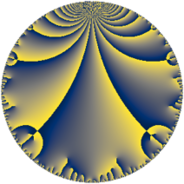# Properties

 Label 21.7.hLevel $21$ Weight $7$ Character orbit 21.h Rep. character $\chi_{21}(2,\cdot)$ Character field $\Q(\zeta_{6})$ Dimension $28$ Newform subspaces $1$ Sturm bound $18$ Trace bound $0$

# Related objects

## Defining parameters

 Level: $$N$$ $$=$$ $$21 = 3 \cdot 7$$ Weight: $$k$$ $$=$$ $$7$$ Character orbit: $$[\chi]$$ $$=$$ 21.h (of order $$6$$ and degree $$2$$) Character conductor: $$\operatorname{cond}(\chi)$$ $$=$$ $$21$$ Character field: $$\Q(\zeta_{6})$$ Newform subspaces: $$1$$ Sturm bound: $$18$$ Trace bound: $$0$$

## Dimensions

The following table gives the dimensions of various subspaces of $$M_{7}(21, [\chi])$$.

Total New Old
Modular forms 36 36 0
Cusp forms 28 28 0
Eisenstein series 8 8 0

## Trace form

 $$28 q - q^{3} + 382 q^{4} - 356 q^{6} + 1120 q^{7} + 1031 q^{9} + O(q^{10})$$ $$28 q - q^{3} + 382 q^{4} - 356 q^{6} + 1120 q^{7} + 1031 q^{9} - 930 q^{10} - 404 q^{12} + 3632 q^{13} + 5450 q^{15} - 13706 q^{16} + 1612 q^{18} + 6830 q^{19} - 11333 q^{21} - 27348 q^{22} - 54498 q^{24} - 7640 q^{25} + 13268 q^{27} + 88522 q^{28} + 32380 q^{30} + 69410 q^{31} - 85459 q^{33} - 31344 q^{34} + 399028 q^{36} - 71830 q^{37} + 104570 q^{39} + 230250 q^{40} - 467782 q^{42} - 498904 q^{43} - 85375 q^{45} - 342660 q^{46} - 324728 q^{48} + 395668 q^{49} + 42177 q^{51} + 218000 q^{52} - 402122 q^{54} + 231660 q^{55} + 786782 q^{57} + 459522 q^{58} + 1021150 q^{60} + 153158 q^{61} + 179011 q^{63} - 1399412 q^{64} + 80896 q^{66} - 977098 q^{67} - 1520742 q^{69} - 139650 q^{70} - 1001352 q^{72} - 488350 q^{73} + 1434610 q^{75} + 6196280 q^{76} + 245176 q^{78} + 380762 q^{79} + 893531 q^{81} - 3665004 q^{82} + 1231496 q^{84} - 912540 q^{85} + 1452416 q^{87} - 1522482 q^{88} - 11713820 q^{90} - 1529416 q^{91} - 1690071 q^{93} - 3034044 q^{94} + 7549346 q^{96} + 7212176 q^{97} + 435866 q^{99} + O(q^{100})$$

## Decomposition of $$S_{7}^{\mathrm{new}}(21, [\chi])$$ into newform subspaces

Label Dim $A$ Field CM Traces $q$-expansion
$a_{2}$ $a_{3}$ $a_{5}$ $a_{7}$
21.7.h.a $28$ $4.831$ None $$0$$ $$-1$$ $$0$$ $$1120$$